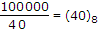# Electronics and Communication Engineering - Digital Electronics

21.

Binary number 11001 is equivalent to decimal number

 A. 35 B. 15 C. 105 D. 25

Explanation:

11001 = 16 + 8 + 1 = 25 in decimal.

22.

Which of the following are included in the architecture of computer?

1. Addressing mode, design of CPU
2. Instruction set, data format
3. Secondary memory, operating system
Select the correct answer using the codes given below:

 A. 1 and 2 B. 2 and 3 C. 1 and 3 D. 1, 2 and 3

Explanation:

Addressing mode, CPU design, Data formats, instruction set are part of Computer Architecture.

23.

Assertion (A): Tristate logic is used for bus oriented systems

Reason (R): The outputs of a tristate logic are 0, 1 and indeterminant.

 A. Both A and R are correct and R is correct explanation of A B. Both A and R are correct but R is not correct explanation of A C. A is true, R is false D. A is false, R is true

Explanation:

Outputs of tristate logic are 0, 1 and high impedance state.

24.

The value of 25 in octal system is

 A. 40 B. 20 C. 100 D. 200

Explanation:

25 in decimal = 32

(32)10 = (100000)2=.

25.

Assertion (A): ECL gate has very high speed of operation.

Reason (R): Transistors in ECL do not go into saturation region.

 A. Both A and R are correct and R is correct explanation of A B. Both A and R are correct but R is not correct explanation of A C. A is true, R is false D. A is false, R is true